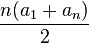# Tag Archives: Java

## Hello world program in Java

Programming in Java has always been fun for me. With this post I begin the Java programming tutorials journey. In this post, we will learn how to write a simple Hello world program in Java. We will learn basic structure of a Java program, how to write, compile and execute a Java program.

## Project Euler 2: Even Fibonacci numbers

### Problem:

Each new term in the Fibonacci sequence is generated by adding the previous two terms. By starting with 1 and 2, the first 10 terms will be:

1, 2, 3, 5, 8, 13, 21, 34, 55, 89, ...

By considering the terms in the Fibonacci sequence whose values do not exceed four million, find the sum of the even-valued terms.
This problem was originally featured on Project Euler.

### Algorithm:

Here we only need to learn how to compute Fibonacci numbers.

### Program in Java:

`/* * Copyright (C) 2015 Pankaj @ http://codeforwin.blogspot.com/ * * This program is free software: you can redistribute it and/or modify * it under the terms of the GNU General Public License as published by * the Free Software Foundation, either version 3 of the License, or * (at your option) any later version. * * This program is distributed in the hope that it will be useful, * but WITHOUT ANY WARRANTY; without even the implied warranty of * MERCHANTABILITY or FITNESS FOR A PARTICULAR PURPOSE.  See the * GNU General Public License for more details. * * You should have received a copy of the GNU General Public License * along with this program.  If not, see <http://www.gnu.org/licenses/>. *//** * * @author Pankaj */public class ProjectEuler2 {        public static void main(String args[] ){        long a,b,c, sum = 0L;        a = 0;        b = 0;        c = 1;                while(c<=4000000) {                        if(c%2==0)                sum += c;                        a = b;            b = c;            c = a+b;        }        System.out.println(sum);    }}`

Happy coding 😉

## Project Euler 1: Multiples of 3 and 5

### Problem:

If we list all the natural numbers below 10 that are multiples of 3 or 5, we get 3, 5, 6 and 9. The sum of these multiples is 23.

We need to find the sum of all the multiples of 3 or 5 below 1000.
View this problem on Project Euler.

### Algorithm:

The problem sounds easy and any beginner can solve this in just few minutes.
What we need to do is:
Step 1: Begin a loop from 3 to 1000
Step 2: If the number is either divisible by 3 or 5 add it to sum.

That's it we need to do. But this algorithm works superbly fine on short data range, but when the data range goes beyond 109 the program takes minutes and hours to finish processing. Hence we need an optimal solution to the problem. And the solution lies in the 10 class basic mathematics(Arithmetic Progression).

Optimal Algorithm:
Here the basic concepts of Arithmetic Progression is used. We need to calculate sum of n elements.

#### Formula:Where n is the number of elements, a1 is the starting element, and an is the last element.

Step 1: Compute sum of all elements under 1000 which are divisible by 3
Step 2: Compute sum of all elements under 1000 which are divisible by 5
Step 3: Compute sum of all elements under 1000 which are divisible by 15
Step 4: Add sum1 + sum2 and subtract from sum3 i.e. sum1 + sum2 - sum3 (Where sum1=Sum of all elements divisible by 3, sum2=Sum of all elements divisible by 5, sum3=Sum of all elements divisible by 15) and we are done.

### Program:

```/*
* Copyright (C) 2015 PankajPrakashh @ http://codeforwin.blogspot.com/
*
* This program is free software: you can redistribute it and/or modify
* the Free Software Foundation, either version 3 of the License, or
* (at your option) any later version.
*
* This program is distributed in the hope that it will be useful,
* but WITHOUT ANY WARRANTY; without even the implied warranty of
* MERCHANTABILITY or FITNESS FOR A PARTICULAR PURPOSE.  See the
* GNU General Public License for more details.
*
* You should have received a copy of the GNU General Public License
* along with this program.  If not, see http://www.gnu.org/licenses/
*/

import java.util.Scanner;

/**
*
* @author Pankaj
*/
public class ProjectEuler1 {

public static void main(String args[]) {
Scanner in = new Scanner(System.in);
long n = in.nextLong();
n--; //Since we need to compute sum below n.

long sum = 0;
long totalElements = 0;

//Check if n is more than or equal to 3 then compute sum of all elements
//divisible by 3 and add to sum.
if(n>=3) {
totalElements = n/3;
sum += (totalElements * ( 3 + totalElements*3)) / 2;
}

//Check if n is more than or equal to 5 then compute sum of all elements
//divisible by 5 and add to sum.
if(n >= 5) {
totalElements = n/5;
sum += (totalElements * (5 + totalElements * 5)) / 2;
}

//Check if n is more than or equal to 15 then compute sum of all elements
//divisible by 15 and subtract from sum.
if(n >= 15) {
totalElements = n/15;
sum -= (totalElements * (15 + totalElements * 15)) / 2;
}

System.out.println(sum);
}
}
```

Happy coding 😉